# 1755 Searching for Prime Decades

### Today’s Puzzle:

Charles Sanders got me thinking about prime decades and how to find them. What a great puzzle for me to work on!

A prime decade is a set of four prime numbers that look the same except for their last digit.

The first decade has 4 prime numbers, 2, 3, 5, 7, but it is NOT a prime decade because 1 and 9 are not prime numbers. 5, 7, 11, 13 is the only prime quadruplet that is not also a prime decade. The first prime decade is 11, 13, 17, 19.

Could 1751, 1753, 1757, and 1759 form a prime decade? Since 1755 is divisible by 15, none of those other four odd numbers can be divisible by 3 or 5, so it is worth looking into if they are all prime numbers. We don’t have to test all four numbers, however, we only need to test 1759 using modular arithmetic. If we note the remainder when we divide 1759 by each prime number from 7 to 41, we will know. (We only have to go to 41 because √1759 < 43, so we don’t need any prime number divisor greater than or equal to 43.)

Will any remainder be a red flag? Yes, if any remainder is 0, 2, 6, or 8, then one of those four odd numbers won’t be prime. Also, if we divide 1759 by 7 and get a remainder of 1, then 1758 and 1751 will both be divisible by 7, and we won’t have a prime decade.

I did the divisions and everything looked great except
1759 ÷ 7 has a remainder of 2, so 1759 – 2 or 1757 is divisible by 7, and
1759 ÷ 17 has a remainder of 8, so 1759 – 8 or 1751 is divisible by 17.

In general, to find prime decades we only need to test the last number of the decades and we only need to test decades if the last number minus 4 is divisible by 15. I made a chart showing only those last numbers, and they appear in the first column. The top two rows are the prime number divisors and their squares. We will divide the last number in the decades by the prime numbers whose square is less than that last number. The remainders in pink are red flags. That would be a 1 in the 7’s column, and 0, 2, 6, or 8 in any column. Any of those would disqualify a decade from being a prime decade. 🙁

The green last numbers (in the first column) show the last number of actual prime decades. 🙂
The yellow last numbers have only ONE divisor that disqualifies the decade. 😐

What are the prime decades in this first millennia?As you can see, decades ending in 19, 109, 199, and 829 are all prime decades. This chart can also be useful in finding prime triplets because at least two of the prime numbers in a prime triplet whose first prime is greater than 10 must come from a decade whose middle number is divisible by 15. If a decade has two prime triplets in it that meet that qualification, it is also a prime decade.

In the next graphic, we see that the decades ending in 1489 and 1879 are prime decades. Here we also test the decade in which our post number, 1755, appears. We see 1759 has remainders of 2 and 8, confirming that 1757 and 1751 are not prime numbers.There is only one prime decade, 2081, 2083, 2087, 2089, in the next set of decades, and only one other decade that even came close to being a second one.There are two prime decades in the next group and several close calls:None in the next group, but there are several decades that ALMOST qualified.There’s a prime decade is in this group as well as a handful of decades that almost qualified.In this next group, there are only two decades that even came close to qualifying.The next two millennia have no prime decades but they do have several decades that came close to qualifying.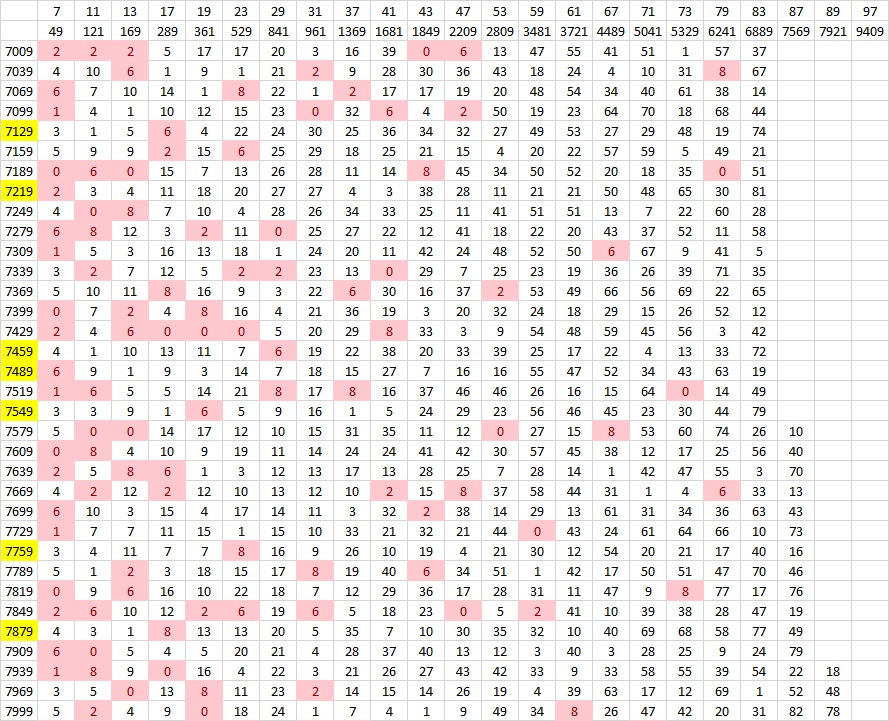Finally, we get another prime decade in this next group! (We would have had one right away if only 9047 wasn’t divisible by 83.)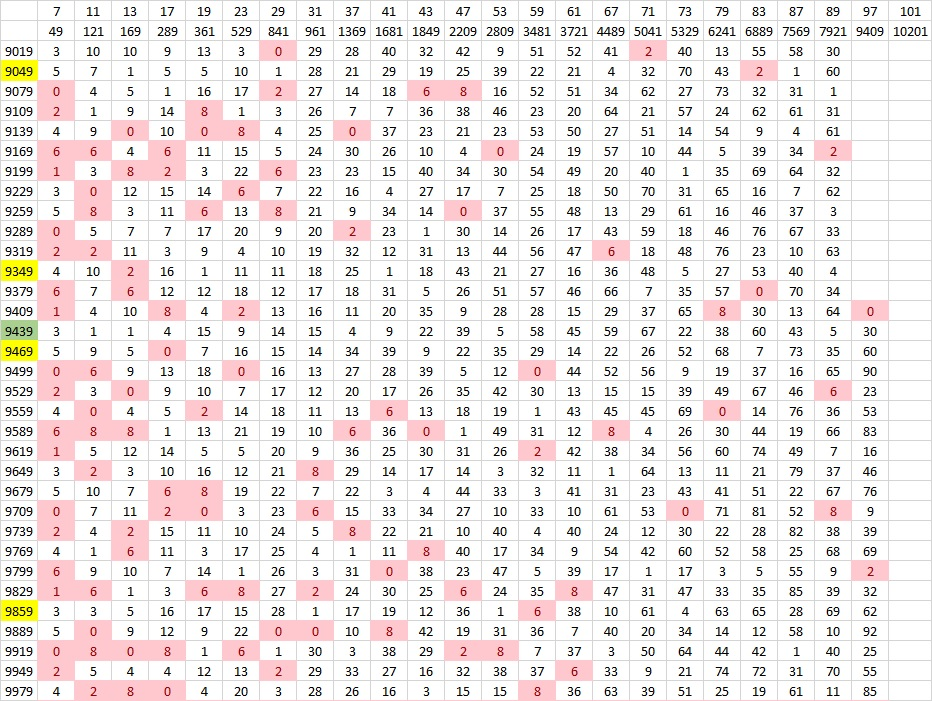I could go on literally forever, but I’ll stop now. Instead, I’ll write a little more about the post number, 1755.

### Factors of 1755:

• 1755 is a composite number.
• Prime factorization: 1755 = 3 × 3 × 3 × 5 × 13, which can be written 1755 = 3³ × 5 × 13.
• 1755 has at least one exponent greater than 1 in its prime factorization so √1755 can be simplified. Taking the factor pair from the factor pair table below with the largest square number factor, we get √1755 = (√9)(√195) = 3√195.
• The exponents in the prime factorization are 3,1 and 1. Adding one to each exponent and multiplying we get (3 + 1)(1 + 1) (1 + 1) = 4 × 2 × 2 = 16. Therefore 1755 has exactly 16 factors.
• The factors of 1755 are outlined with their factor pair partners in the graphic below.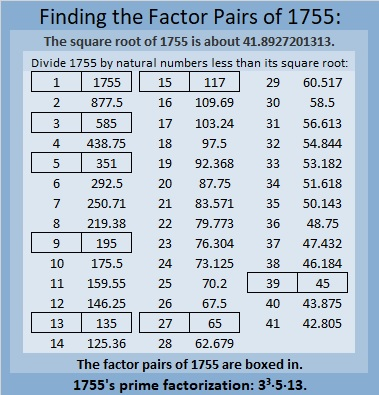### More About the Number 1755:

1755 is the hypotenuse of FOUR Pythagorean triples:
432-1701-1755, which is 27 times (16-63-65)
675-1620-1755, which is (5-12-13) times 135,
891-1512-1755, which is 27 times (33-56-65), and
1053-1404-1755, which is (3-4-5) times 351.

# 1735 What Amazing Mathematical Pattern I Noticed Today!

### Today’s Puzzle:

Will the common factor of 60 and 10 be 5 or will it be 10? Don’t guess. There is a better place to start this puzzle. After using logic to find some of the other factors, you will know if you should use 5 or 10. Don’t let me trip you up!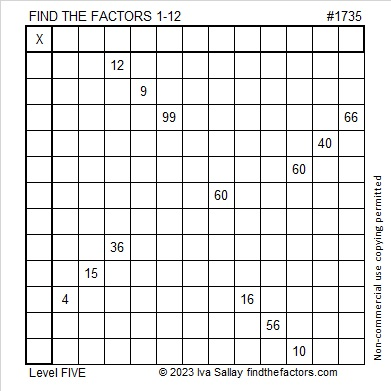### Factors of 1735:

• 1735 is a composite number.
• Prime factorization: 1735 = 5 × 347.
• 1735 has no exponents greater than 1 in its prime factorization, so √1735 cannot be simplified.
• The exponents in the prime factorization are 1 and 1. Adding one to each exponent and multiplying we get (1 + 1)(1 + 1) = 2 × 2 = 4. Therefore 1735 has exactly 4 factors.
• The factors of 1735 are outlined with their factor pair partners in the graphic below.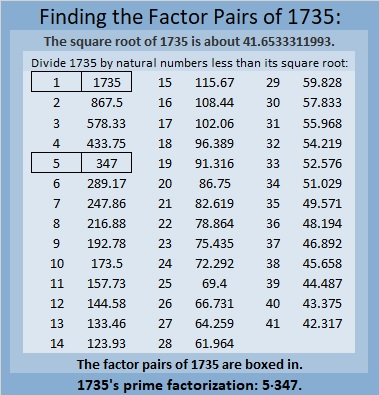### More About the Number 1735 and an Amazing Pattern:

1735 is the hypotenuse of a Pythagorean triple:
1041-1388-1735, which is (3-4-5) times 347.

1735 is 567 in base 18 because
5(18²) + 6(18) + 7(1) = 1735.

I know neither of those facts is amazing, but read on:

1735 is the sum of two, five, and ten consecutive positive numbers:
867 + 868 = 1735,
345 + 346 + 347 + 348 + 349 = 1735, and
169 + 170 + 171 + 172 + 173 + 174 + 175 + 176 + 177 + 178 = 1735.

1735 is the difference of two squares in two different ways:
868² – 867² = 1735, and
176² – 171² = 1735.

That first difference of two squares used the two consecutive numbers I listed above in red. Since mathematics is the study of patterns, I wondered if there would also be a relationship of squares with the ten consecutive numbers in blue. I typed into my calculator:
178² – 177² + 176² – 175² + 174² – 173² + 172² – 171² + 170² – 169² =

Guess what the answer was? If you guessed 1735, you would be right! Oh, mathematics is certainly filled with amazing patterns! I’m thrilled that I spotted this one! It appears something similar can be written whenever a number is the sum of an even amount of consecutive numbers! I find that amazing!

I also noticed that the second difference of two squares noted above is contained in the string. I underlined those two squares. Since the difference of those squares by themselves equals the whole string, it follows that the rest of those squares added and subtracted equal zero. That means the sum of the rest of the positive terms must equal the sum of the rest of the negative terms:
178² + 174² + 172² + 170² = 120144 = 177² + 175² + 173² + 169².
I’ve never found a string of four squares equal to another string of four squares before, so naturally, I’m excited! I’m anxious to apply this pattern to other numbers that are the sum of ten consecutive numbers. For example, the first ten numbers add up to 55.
10² – 9² + – 7² + 6² – 5² + 4² – 3² + 2² – 1² =55. And since 8² – 3² = 55, it follows that
10²  + 6² + 4² + 2² = 156 = 9² + 7² + 5²+ 1².
It is no longer “impossible” for me to find these equal sums! Here’s another that connects this year with other recent years:We can all hope this equation doesn’t predict the future. Ha ha! I’m confident it is NOT a bad omen!